NEET  >  31 Year NEET Previous Year Questions: Gravitation - 2

# 31 Year NEET Previous Year Questions: Gravitation - 2

Test Description

## 30 Questions MCQ Test Physics Class 11 | 31 Year NEET Previous Year Questions: Gravitation - 2

31 Year NEET Previous Year Questions: Gravitation - 2 for NEET 2023 is part of Physics Class 11 preparation. The 31 Year NEET Previous Year Questions: Gravitation - 2 questions and answers have been prepared according to the NEET exam syllabus.The 31 Year NEET Previous Year Questions: Gravitation - 2 MCQs are made for NEET 2023 Exam. Find important definitions, questions, notes, meanings, examples, exercises, MCQs and online tests for 31 Year NEET Previous Year Questions: Gravitation - 2 below.
Solutions of 31 Year NEET Previous Year Questions: Gravitation - 2 questions in English are available as part of our Physics Class 11 for NEET & 31 Year NEET Previous Year Questions: Gravitation - 2 solutions in Hindi for Physics Class 11 course. Download more important topics, notes, lectures and mock test series for NEET Exam by signing up for free. Attempt 31 Year NEET Previous Year Questions: Gravitation - 2 | 30 questions in 60 minutes | Mock test for NEET preparation | Free important questions MCQ to study Physics Class 11 for NEET Exam | Download free PDF with solutions
 1 Crore+ students have signed up on EduRev. Have you?
31 Year NEET Previous Year Questions: Gravitation - 2 - Question 1

### The distance of two planets from the sun are 1013 and 1012 metres respectively. The ratio of time periods of these two planets is 

Detailed Solution for 31 Year NEET Previous Year Questions: Gravitation - 2 - Question 1

T2 ∝ R3 (Kepler's law)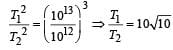31 Year NEET Previous Year Questions: Gravitation - 2 - Question 2

### The largest and the shortest distance of the earth from the sun are r1 and r2. Its distance from the sun when it is at perpendicular to the major-axis of the orbit drawn from the sun is 

Detailed Solution for 31 Year NEET Previous Year Questions: Gravitation - 2 - Question 2

Applying the properties of ellipse, we have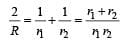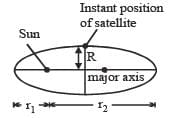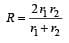31 Year NEET Previous Year Questions: Gravitation - 2 - Question 3

### If the gravitational force between two objects were proportional to 1/R (and not as 1/R2) where R is separation between them, then a particle in circular orbit under such a force would have its orbital speed v proportional to 

Detailed Solution for 31 Year NEET Previous Year Questions: Gravitation - 2 - Question 3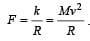Hence v ∝ R0

31 Year NEET Previous Year Questions: Gravitation - 2 - Question 4

For a satellite escape velocity is 11 km/s. If the satellite is launched at an angle of 60° with the vertical, then escape velocity will be 

Detailed Solution for 31 Year NEET Previous Year Questions: Gravitation - 2 - Question 4

Since, escape velocity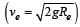is independent of angle of projection, so it will not change.

31 Year NEET Previous Year Questions: Gravitation - 2 - Question 5

A planet is moving in an elliptical orbit around the sun. If T, V, E and L stand respectively for its kinetic energy, gravitational potential energy, total energy and magnitude of angular momentum about the centre of force, which of the following is correct ? 

Detailed Solution for 31 Year NEET Previous Year Questions: Gravitation - 2 - Question 5

In a circular or elliptical orbital motion, torque is always acting parallel to displacement or velocity. So, angular momentum is conserved.
In attractive field, potential energy is negative.
Kinetic energy changes as velocity increase when distance is less. So, option (c) is correct.

31 Year NEET Previous Year Questions: Gravitation - 2 - Question 6

A satellite of mass m is orbiting around the earth in a circular orbit with a velocity v. What will be its total energy? 

Detailed Solution for 31 Year NEET Previous Year Questions: Gravitation - 2 - Question 6

Total energy =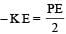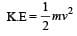31 Year NEET Previous Year Questions: Gravitation - 2 - Question 7

A seconds pendulum is mounted in a rocket. Its period of oscillation decreases when the rocket 

Detailed Solution for 31 Year NEET Previous Year Questions: Gravitation - 2 - Question 7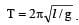. When the rocket accelerates upwards g increases to ( g + a).

31 Year NEET Previous Year Questions: Gravitation - 2 - Question 8

The mean radius of earth is R, its angular speed on its own axis is ω and the acceleration due to gravity at earth's surface is g. What will be the radius of the orbit of a geostationary satellite ? 

Detailed Solution for 31 Year NEET Previous Year Questions: Gravitation - 2 - Question 8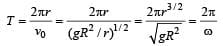Hence,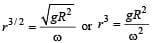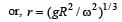31 Year NEET Previous Year Questions: Gravitation - 2 - Question 9

A satellite A of mass m is at a distance of r from the surface of the earth. Another satellite B of mass 2m is at a distance of 2r from  the earth’s centre. Their time periods are in the ratio of 

Detailed Solution for 31 Year NEET Previous Year Questions: Gravitation - 2 - Question 9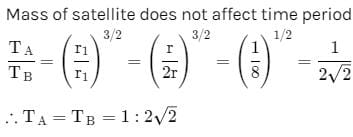31 Year NEET Previous Year Questions: Gravitation - 2 - Question 10

The escape velocity from earth is 11.2 km/s. If a body is to be projected in a direction making an angle 45° to the vertical, then the escape velocity is

Detailed Solution for 31 Year NEET Previous Year Questions: Gravitation - 2 - Question 10

Escape velocity does not depend on the angle of projection.

31 Year NEET Previous Year Questions: Gravitation - 2 - Question 11

The distance of Neptune and Saturn from the sun is nearly 1013 and 1012 meter respectively.Assuming that they move in circular orbits, their periodic times will be in the ratio 

Detailed Solution for 31 Year NEET Previous Year Questions: Gravitation - 2 - Question 11

T2 ∝ R3 (According to Kepler ’s law)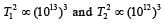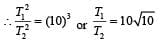31 Year NEET Previous Year Questions: Gravitation - 2 - Question 12

A satellite in force free space sweeps stationary interplanetary dust at a rate dM/dt = αv where M is the mass and v is the velocity of the satellite and α is a constant. What is the deceleration of the satellite? 

Detailed Solution for 31 Year NEET Previous Year Questions: Gravitation - 2 - Question 12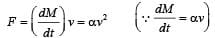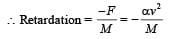31 Year NEET Previous Year Questions: Gravitation - 2 - Question 13

The escape velocity from the surface of the earth is ve.The escape velocity from the surface of a planet whose mass and radius are three times those of the earth, will be 

Detailed Solution for 31 Year NEET Previous Year Questions: Gravitation - 2 - Question 13

Escape velocity on surface of earth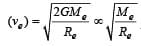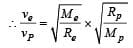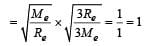or,   vP= ve.

31 Year NEET Previous Year Questions: Gravitation - 2 - Question 14

What will be the formula of the mass in terms of g, R and G (R = radius of earth) 

Detailed Solution for 31 Year NEET Previous Year Questions: Gravitation - 2 - Question 14

We know that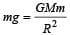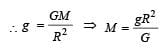31 Year NEET Previous Year Questions: Gravitation - 2 - Question 15

A ball is dropped from a satellite revolving around the earth at a height of 120 km. The ball will 

Detailed Solution for 31 Year NEET Previous Year Questions: Gravitation - 2 - Question 15

The orbital speed of satellite is independent of mass of satellite, so the ball will behave as a satellite and will continue to move with the same speed in the original orbit.

31 Year NEET Previous Year Questions: Gravitation - 2 - Question 16

The period of revolution of planet A around the Sun is 8 times that of B. The distance of A from the Sun is how many times greater than that of B from the Sun? 

Detailed Solution for 31 Year NEET Previous Year Questions: Gravitation - 2 - Question 16

(a) : Period of revolution of planet   A(TA)=8TB
According to Kepler's III law of planetary motion
T2∝R3

Therefore;

=> (rA / rB)= (TA / TB)2

=> (8TA / TB)2 = 64

=> rA / rB = 4

or  r= 4 rB

31 Year NEET Previous Year Questions: Gravitation - 2 - Question 17

The escape velocity of a body on the surface of the earth is 11.2 km/s. If the earth’s mass increases to twice its present value and the radius of the earth becomes half, the escape velocity would become 

Detailed Solution for 31 Year NEET Previous Year Questions: Gravitation - 2 - Question 17

Escape velcocity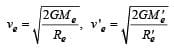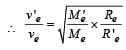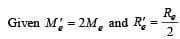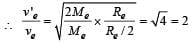v 'e = 2ve = 2 x 11.2 = 22.4 km/s

31 Year NEET Previous Year Questions: Gravitation - 2 - Question 18

The escape velocity of a sphere of mass m is given by (G = Universal gravitational constant; M = Mass of the earth and Re = Radius of the earth)     

Detailed Solution for 31 Year NEET Previous Year Questions: Gravitation - 2 - Question 18

Escape velocity is the minimum velocity with which a body is projected to escape from earth's gravitational field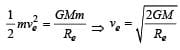31 Year NEET Previous Year Questions: Gravitation - 2 - Question 19

The escape velocity on the surface of earth is 11.2 km/s. What would be the escape velocity on the surface of another planet of the same mass but 1/4 times the radius of the earth? 

Detailed Solution for 31 Year NEET Previous Year Questions: Gravitation - 2 - Question 19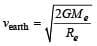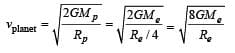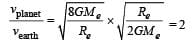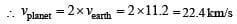31 Year NEET Previous Year Questions: Gravitation - 2 - Question 20

A body weighs 72 N on the surface of the earth.What is the gravitational force on it due to earth at a height equal to half the radius of the earth from the surface? 

Detailed Solution for 31 Year NEET Previous Year Questions: Gravitation - 2 - Question 20

mg = 72 N  (body weight on the surface)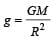At a height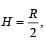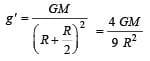Body weight at height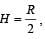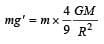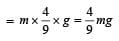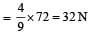31 Year NEET Previous Year Questions: Gravitation - 2 - Question 21

Assuming earth to be a sphere of uniform density, what is the value of ‘g’ in a mine 100 km below the earth’s surface? (Given, R = 6400 km) 

Detailed Solution for 31 Year NEET Previous Year Questions: Gravitation - 2 - Question 21

We know that effective gravity g' at depth below earth surface is given by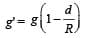Here, d = 100 km, R = 6400 km,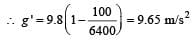31 Year NEET Previous Year Questions: Gravitation - 2 - Question 22

The potential energy of a satellite, having mass m and rotating at a height of 6.4 × 106m from the earth surface, is 

Detailed Solution for 31 Year NEET Previous Year Questions: Gravitation - 2 - Question 22

Mass of the satellite = m and heigh t of satellite from earth (h) = 6.4 × 106 m.
We know that gravitational potential energy of the satellite at height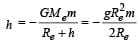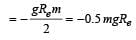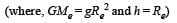31 Year NEET Previous Year Questions: Gravitation - 2 - Question 23

With what velocity should a particle be projected so that its height becomes equal to radius of earth?           

Detailed Solution for 31 Year NEET Previous Year Questions: Gravitation - 2 - Question 23

From conservation of energy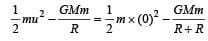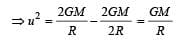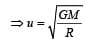31 Year NEET Previous Year Questions: Gravitation - 2 - Question 24

Assuming the radius of the earth as R, the change in gravitational potential energy of a body of mass m, when it is taken from the earth's surface to a height 3R above its surface, is 

Detailed Solution for 31 Year NEET Previous Year Questions: Gravitation - 2 - Question 24

Gravitational potential energy (GPE) on the surface of earth,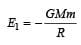GPE at 3R,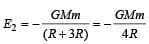∴ Change in GPE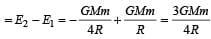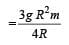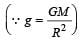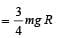31 Year NEET Previous Year Questions: Gravitation - 2 - Question 25

The acceleration due to gravity on the planet A is 9 times the acceleration due to gravity on planet B. A man jumps to a height of 2m on the surface of A. What is the height of jump by the same person on the planet B? 

Detailed Solution for 31 Year NEET Previous Year Questions: Gravitation - 2 - Question 25

Applying conservation of total mechanical energy principle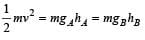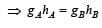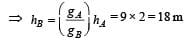31 Year NEET Previous Year Questions: Gravitation - 2 - Question 26

Two spheres of masses m and M are situated in air and the gravitational force between them is F.The space around the masses is now filled with a liquid of specific gravity 3. The gravitational force will now be 

Detailed Solution for 31 Year NEET Previous Year Questions: Gravitation - 2 - Question 26

Gravitational force is independent of medium, Hence, this will remain same.

31 Year NEET Previous Year Questions: Gravitation - 2 - Question 27

The density of a newly discovered planet is twice that of earth. The acceleration due to gravity at the surface of the planet is equal to that at the surface of the earth. If the radius of the earth is R, the radius of the planet would be

Detailed Solution for 31 Year NEET Previous Year Questions: Gravitation - 2 - Question 27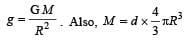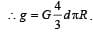At the surface of planet,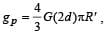At the surface of the earth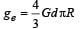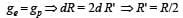31 Year NEET Previous Year Questions: Gravitation - 2 - Question 28

Imagine a new planet having the same density as that of earth but it is 3 times bigger than the earth in size. If the acceleration due to gravity on the surface of earth is g and that on the surface of the new planet is g’, then 

Detailed Solution for 31 Year NEET Previous Year Questions: Gravitation - 2 - Question 28

We know that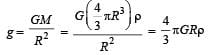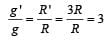∴  g ' = 3g

31 Year NEET Previous Year Questions: Gravitation - 2 - Question 29

For a satellite moving in an or bit ar ound the earth, the ratio of kinetic energy to potential energy is 

Detailed Solution for 31 Year NEET Previous Year Questions: Gravitation - 2 - Question 29

K.E. of satellite moving in an orbit around the earth  is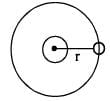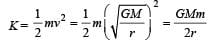P.E. of satellite and earth system is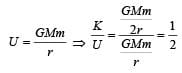31 Year NEET Previous Year Questions: Gravitation - 2 - Question 30

The Earth is assumed to be a sphere of radius R.
A platform is arranged at a height R from the surface of the Earth. The escape velocity of a body from this platform is fv, where v is its escape velocity from the surface of the Earth.
The value of f is 

Detailed Solution for 31 Year NEET Previous Year Questions: Gravitation - 2 - Question 30

Potential energy at height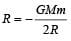If m be the mass of a body which is thrown with velocity ve so that it goes out of gravitational field from distance R, then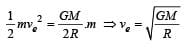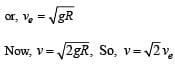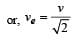Comparing it with given equation,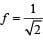## Physics Class 11

127 videos|464 docs|210 tests
 Use Code STAYHOME200 and get INR 200 additional OFF Use Coupon Code
Information about 31 Year NEET Previous Year Questions: Gravitation - 2 Page
In this test you can find the Exam questions for 31 Year NEET Previous Year Questions: Gravitation - 2 solved & explained in the simplest way possible. Besides giving Questions and answers for 31 Year NEET Previous Year Questions: Gravitation - 2, EduRev gives you an ample number of Online tests for practice

## Physics Class 11

127 videos|464 docs|210 tests

### How to Prepare for NEET

Read our guide to prepare for NEET which is created by Toppers & the best Teachers